Part 19: Four integrals defining the 4D complex number tau.

It is a bit late but a happy new year anyway! In this post we will do a classic from the complex plane: calculation of the log of the first imaginary unit.

On the complex plane this is log i and on the complex 4D space this is log l .

Because this number is so important I have given it a separate name a long long time ago: These are the numbers tau in the diverse dimensions. In the complex plane it has no special name and it simply is i times pi/2.

On the real line it is pretty standard to define the log functions as the integral of the inverse 1/x. After all the derivative of log x on the real line is 1/x and as such you simply define the log to be the integral of the derivative…

On the complex plane you can do the same but depending of how your path goes around zero you can get different answers. Also in the complex plane (and other higher dimensional number systems) the log is ‘multi valued’. That is a reflection of the fact we can find exponential periodic functions also known as the exponential circles and curves.

The integrals in this part number 19 on the basics of 4D complex numbers are very hard to crack. I know of no way to find primitives and to crack them that way. May be that is possible, may be it is not, I just do not know. But because I developed the method of matrix diagonals for finding expressions for the value of those difficult looking integrals, more or less in an implicit manner we give the right valuations to those four integrals.

With the word ‘implicit’ I simply mean we skip the whole thing of caculating the number tau via matrix diagonalization. We only calculate what those integrals actually are in terms of a half circle with coordinates cos t and sin t.

This post is 8 pictures long in the usual size of 550 by 775 pixels (I had to enlarge the latest picture a little bit). I hope it is not loaded with typo’s any more and you have a more or less clean mathematical experience: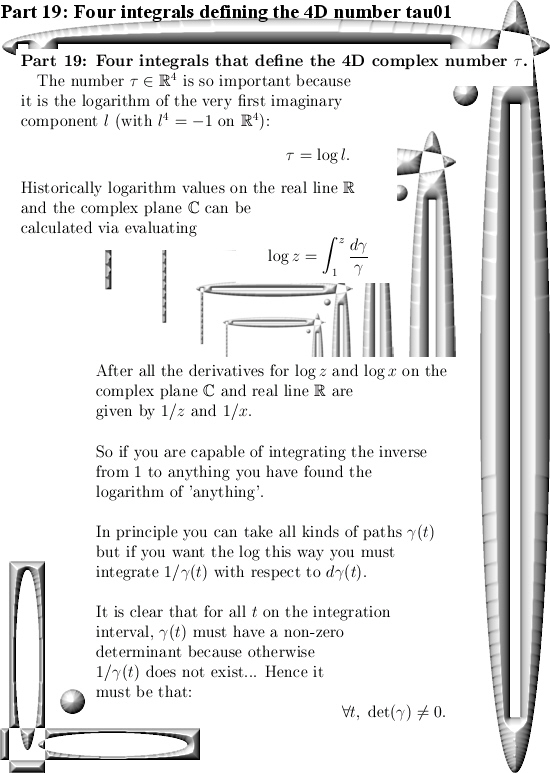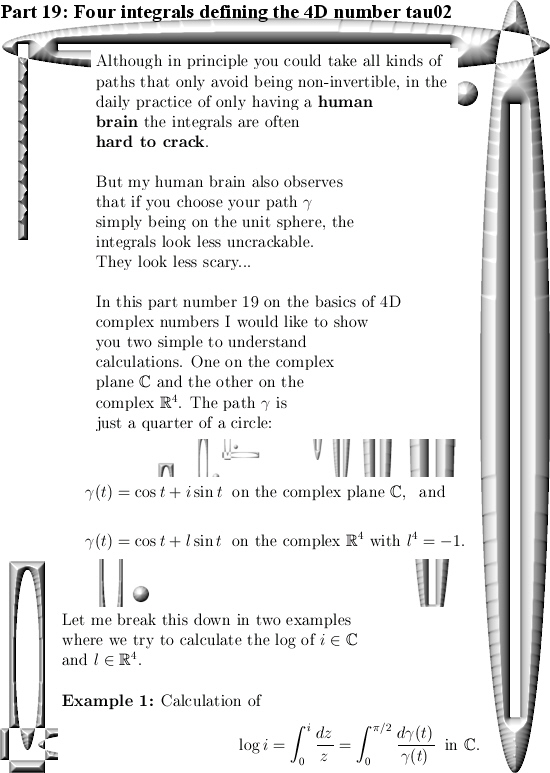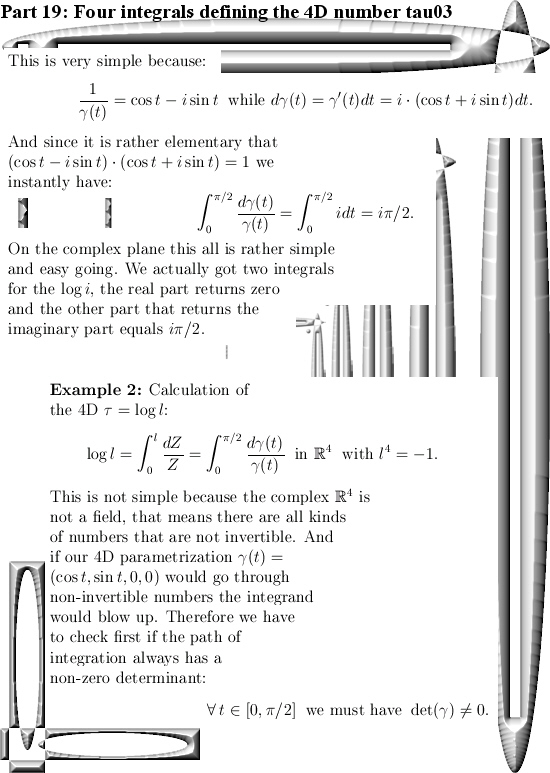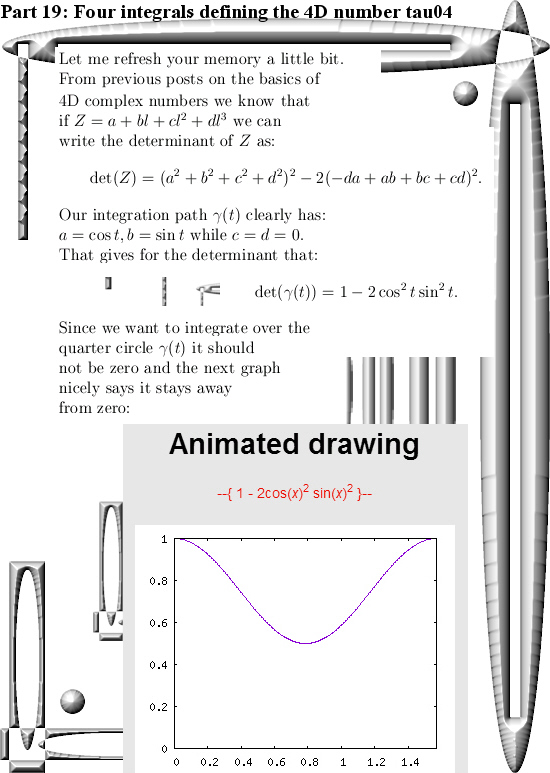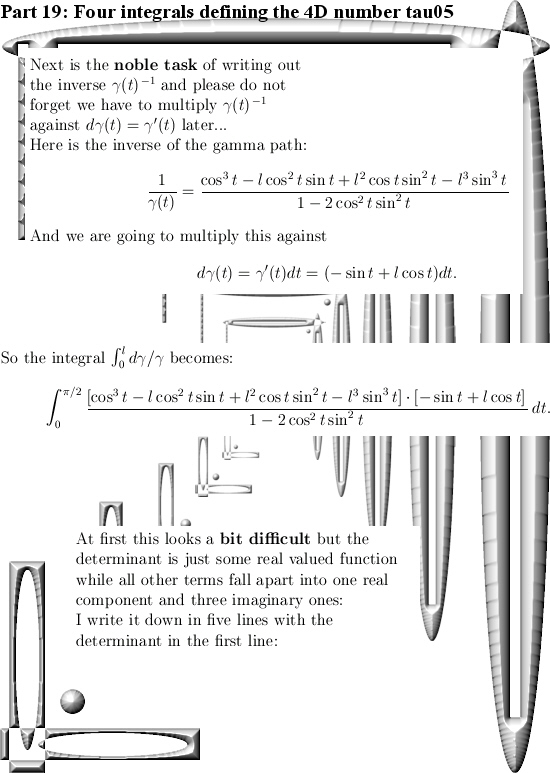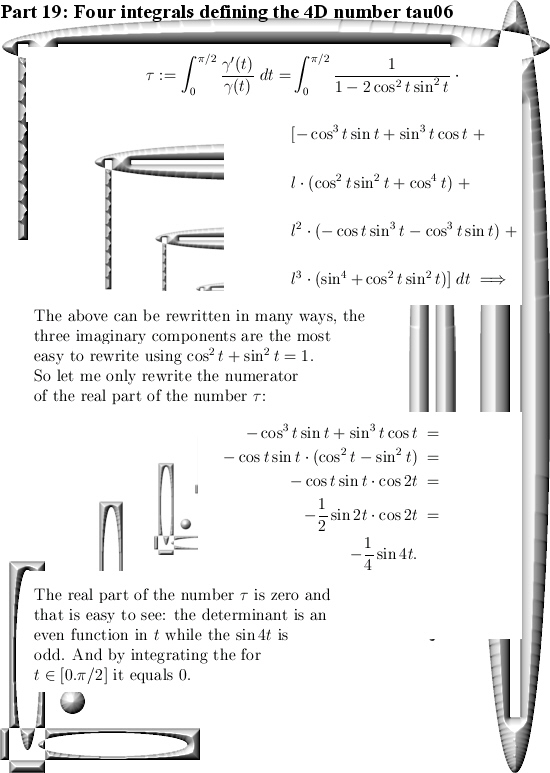End of this post.

Calculation of the 4D number tau diagonal matrix style.

In the begin of this series on basic and elementary calculations you can do with 4D complex numbers we already found what the number tau is. We used stuff like the pull back map… But you can do it also with the method from the previous post about how to find the matrix representation for any 4D complex number Z given the eigenvalues.

Finding the correct eigenvalues for tau is rather subtle, you must respect the behavior of the logarithm function in higher dimensions. It is not as easy as on the real line where you simply have log ab = log a + log b for positive reals a and b.

But let me keep this post short and stop all the blah blah.

Just two nice pictures is all to do the calculation of the 4D complex number tau: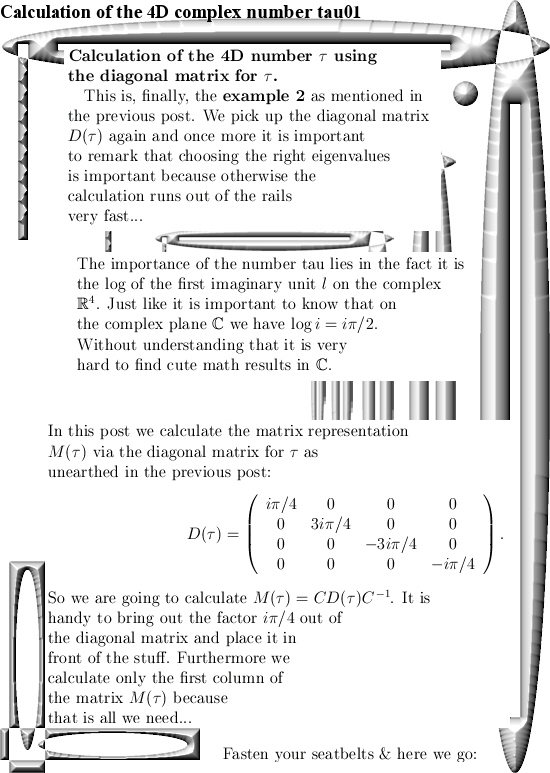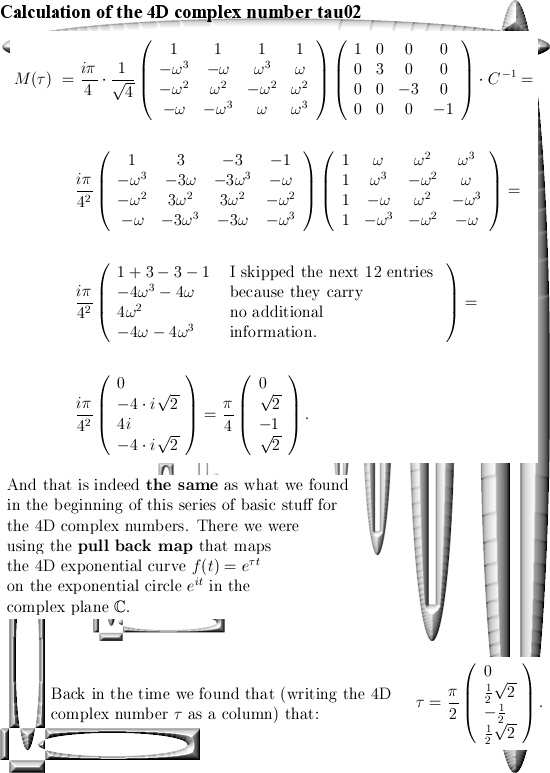(Oops, two days later I repaired a silly typo where I did forget one minus sign. It was just a dumb typo that likely did not lead to much confusion. So I will not take it in the ‘Corrections’ categorie on this website that I use for more or less more significant repairs…)

Ok, that was it.

Diagonal matrices for all 4D complex numbers.

This website is now about 3 years old, the first post was on 14 Nov 2015 and today I hang in with post number 100. That is a nice round number and this post is part 15 in the series known as the Basics for 4D complex numbers.

We are going to diagonalize all those matrix representations M(Z) we have for all 4D complex numbers Z. As a reader you are supposed to know what diagonalization of a matrix actually is, that is in most linear algebra courses so it is widely spread knowledge in the population.

Now at the end of this nine pictures long post you can find how you can calculate the matrix representation for M(l) where l is the first imaginary unit in the 4D complex number system. And I understand that people will ask full of bewilderment, why do this in such a difficult way? That is a good question, but look a bit of the first parts where I gave some examples about how to calculate the number tau that was defined as log l. And one way of doing that was using the pull back map but with matrix diagonalization you have a general method that works in all dimensions.

Beside that this is an all inclusive approach when it comes to the dimension, in practice you can rely on internet applets that use commonly known linear algebra. Now if you are a computer programmer you can automate the process of diagonalization of a matrix. I am very bad in writing computer programs, but if you can write code in an environment where you can do symbolic calculus in your code, it would be handy if that is on such a level you can use the so called roots of unity from the complex plane. After all the eigenvalues you encounter in the 4D complex number system are always based on these roots of unity and the eigenvectors are too…

This post number 100 is 9 pictures long, as usual picture size is 550 x 775 pixels.
In the next post number 101 we will use this method to calculate the matrix representation of the number tau (that is the log of the first imaginary unit l).

Ok, here are the pictures: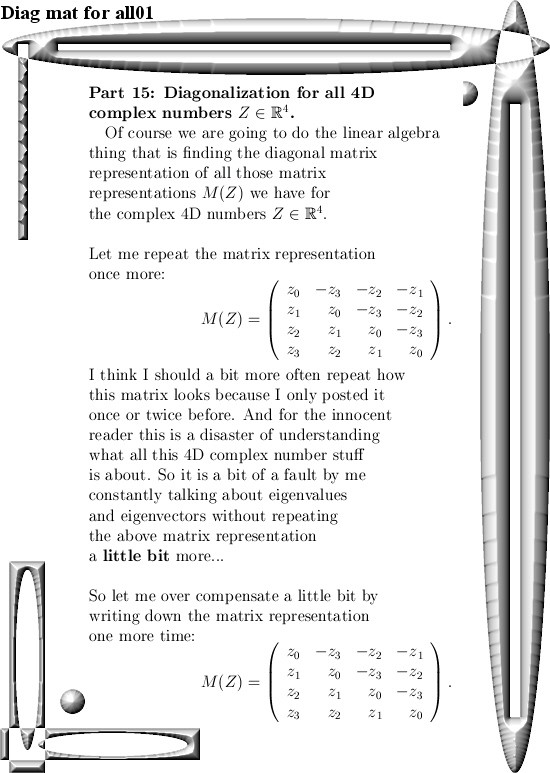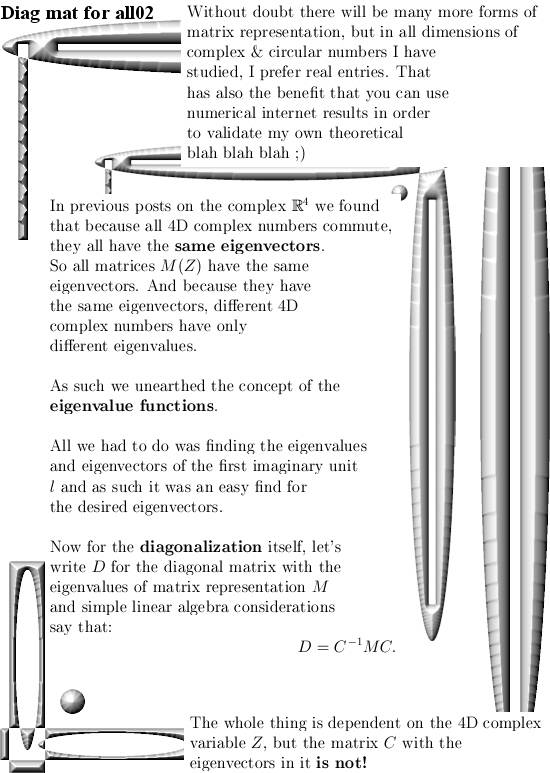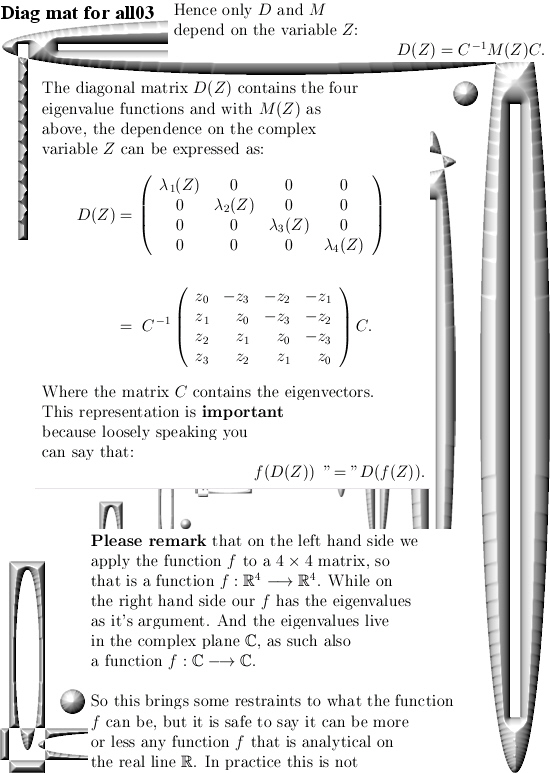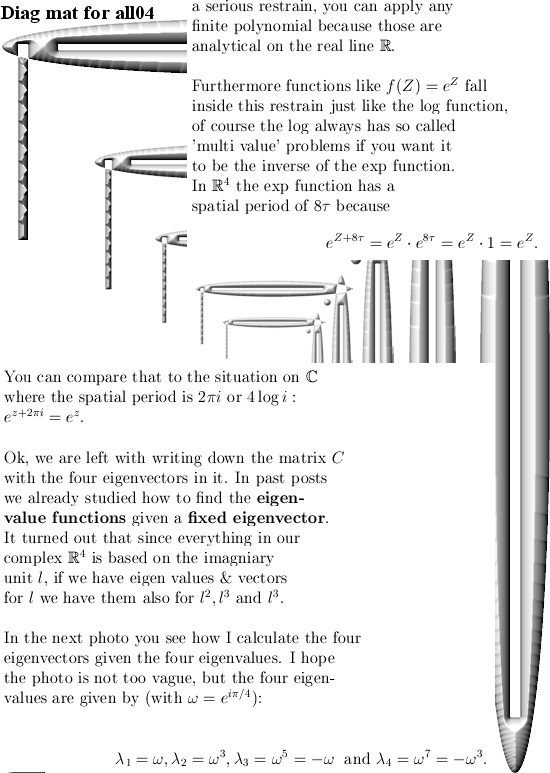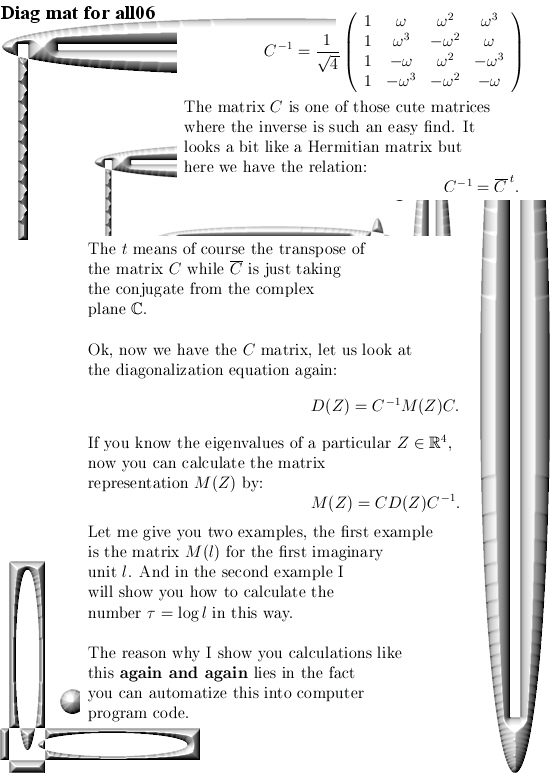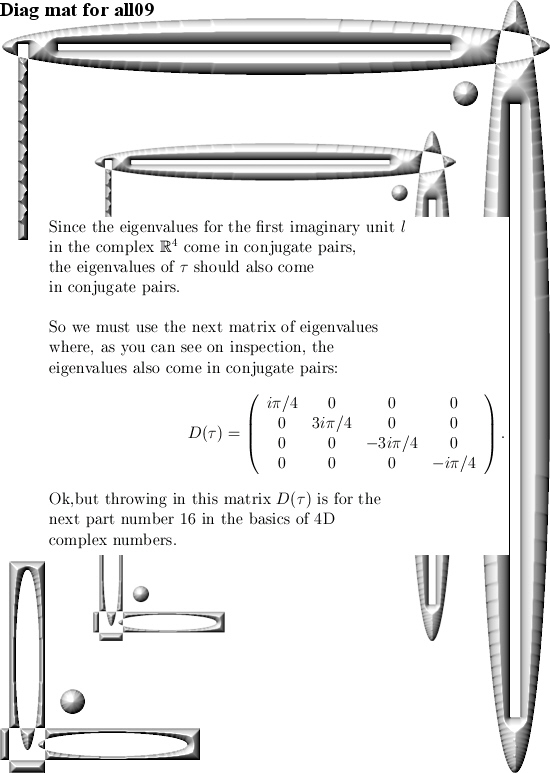That´s it, in the next post we go further with the number tau and from the eigenvalues of tau calculate the matrix representation. So see you around.

Part 14: The Cauchy integral representation for the 4D complex numbers.

It took me longer than expected to craft this update. That is also the nature of the subject; you can view and do math with Cauchy integral representation in many ways. In the end I settled on doing it just for polynomials of finite degree and even more simple: these polynomials are real valued on the real line. (So they have only real coefficients and after that are extended to the space of 4D complex numbers).

In another development, last week we had the yearly circus of Noble prizes and definitely the most cute thing ever was those evolving protein molecules. Because if you can use stuff like the e-coli bacteria you can indeed try if you can (forcefully) evolve the proteins they make… That was like WOW. Later I observed an interview with that chemistry Nobel prize winner and she stated that when she began she was told ‘gentlemen don’t do this kind of thing’.

So she neglected that ‘gentlemen stuff’ and just went on with it. That is a wise thing because if you only do what all those middle age men tell you to do you will find yourself in the very same hole as they are in…
The physics prize was also interesting, for myself speaking I was glad we did not observe those physics men totally not understanding electron spin but with the usual flair of total arrogance keep on talking about spin up and spin down.

You can also turn that spin nonsense upside down: If elementary particles only carry monopole electrical charge than why should electrons be bipolar when it comes to magnetism? That Gauss law of magnetism is only a thing for macroscopic things, there is no experimental proof it holds for quantum particles…

But let’s talk math because this update is not about what I think of electron spin. This is the second Cauchy integral representation I crafted in my life. Now the last years I produced a whole lot of math, my main file is now about 600 pages long. But only that very first Cauchy integral representation is something that I printed out on a beautiful glossy paper of size A0. That first Cauchy integral representation was on the space of 3D numbers and there life is hard: The number tau has determinant zero and as such it is not invertible. But I was able to complexify the 3D circular numbers and it was stunning to understand the number tau in that complexification of the 3D circular numbers. Just stunning…

Therefore I took so much time in trying to find an easy class of functions on the space of 4D complex numbers. I settled for easy to understand polynomials, after all any polynomial gives the same value everywhere if you write them as a Taylor series.
Since this property of polynomials is widely spread I can safely say this in this part 14 of the basics to the 4D complex numbers we have the next Theorem:
THEOREM: The math will do the talking.
PROOF: Just read the next 12 pictures. QED.

As usual all pictures are 550 x 775 pixels in size. I also use a thing I name ‘the heart of the Cauchy integral’, that is not a widely known thing so take your time so that the mathematical parts of your brain can digest it…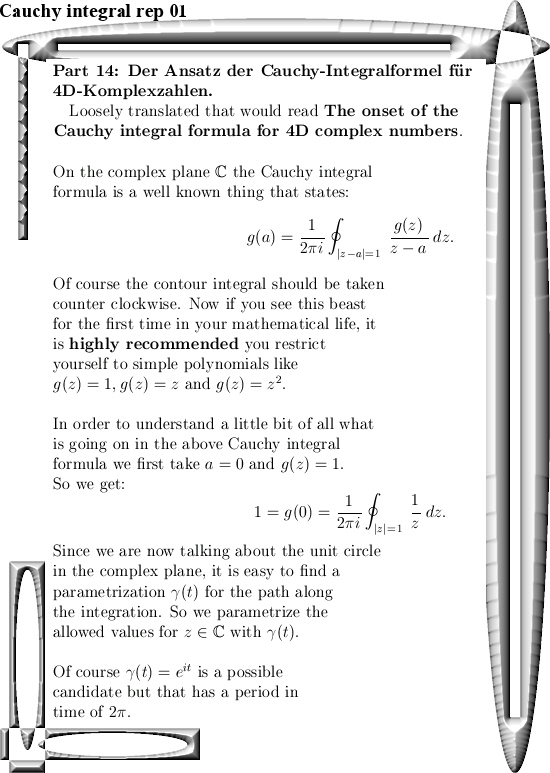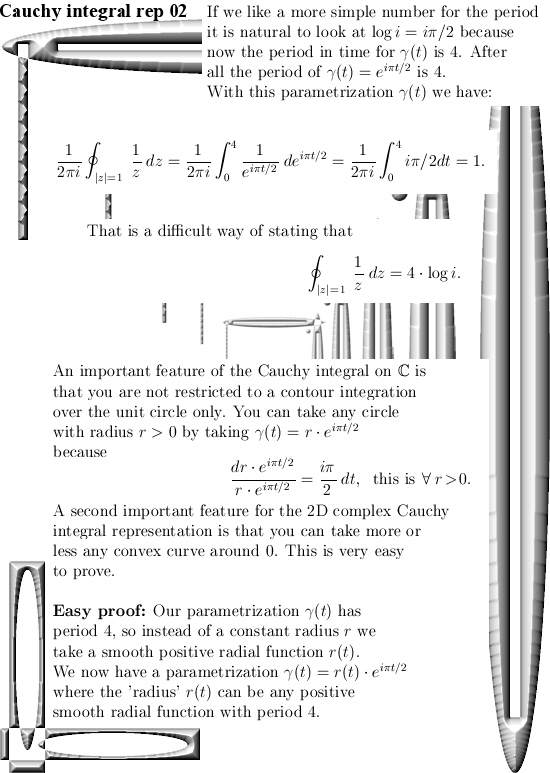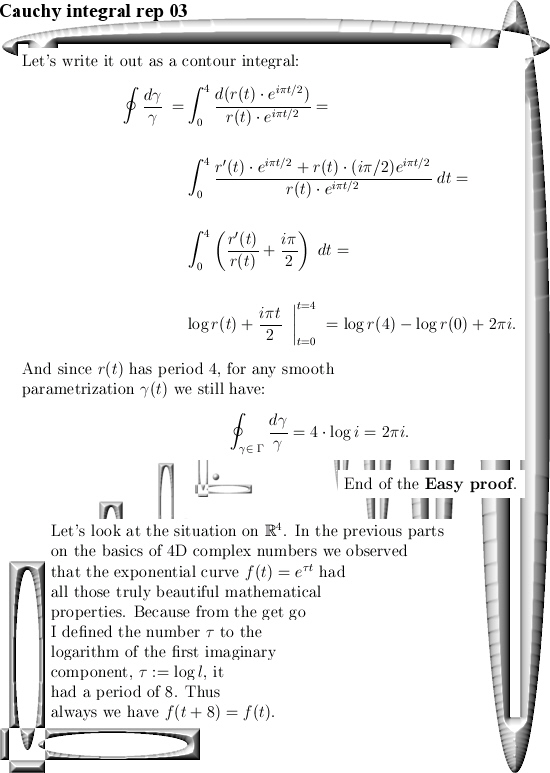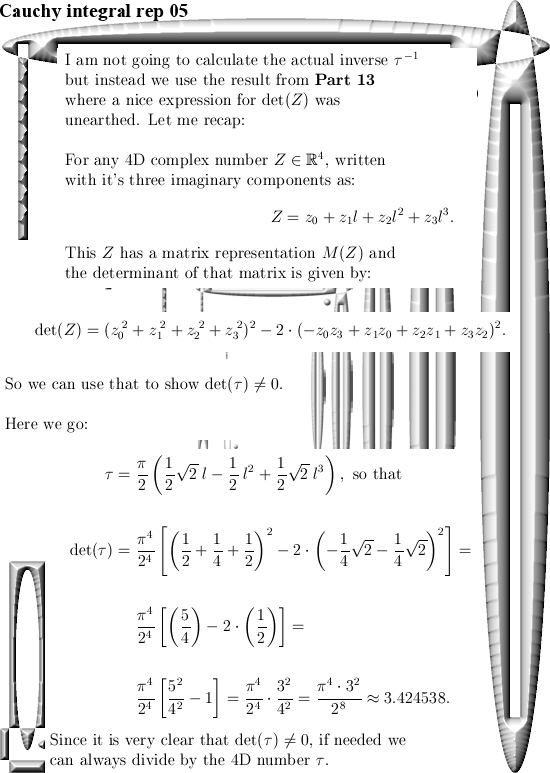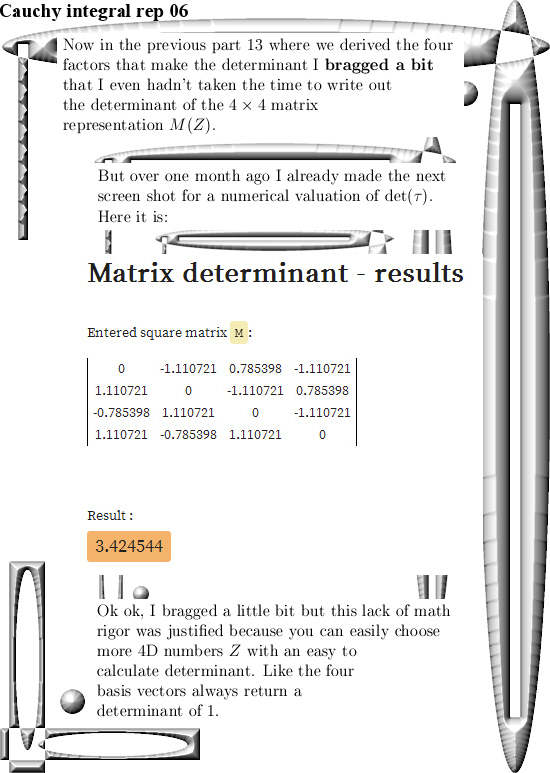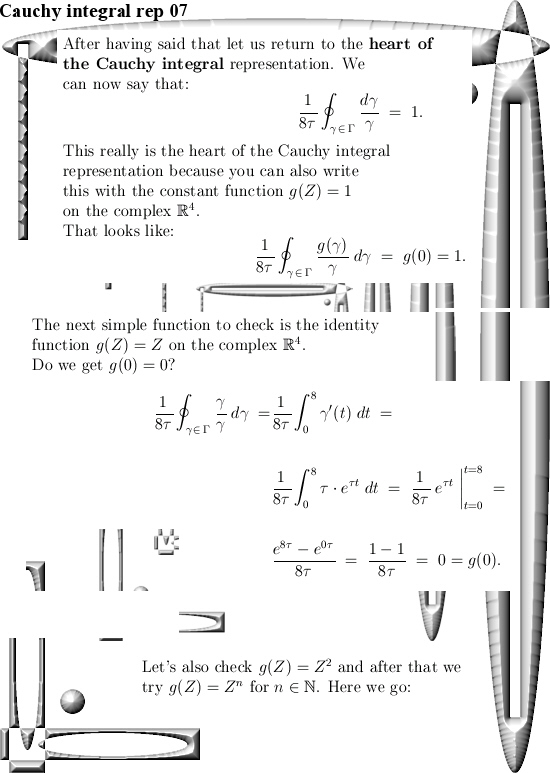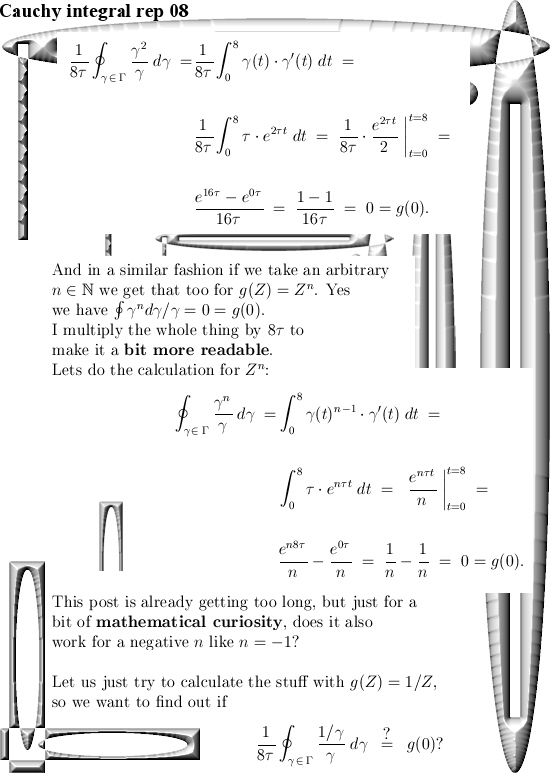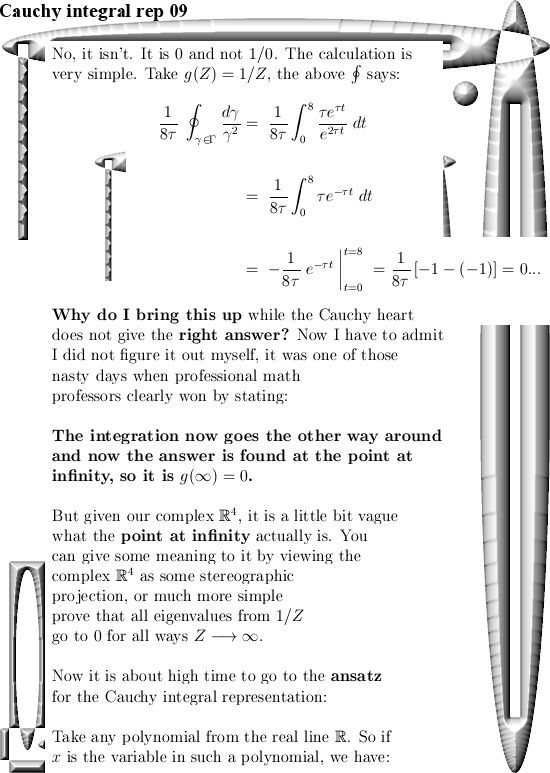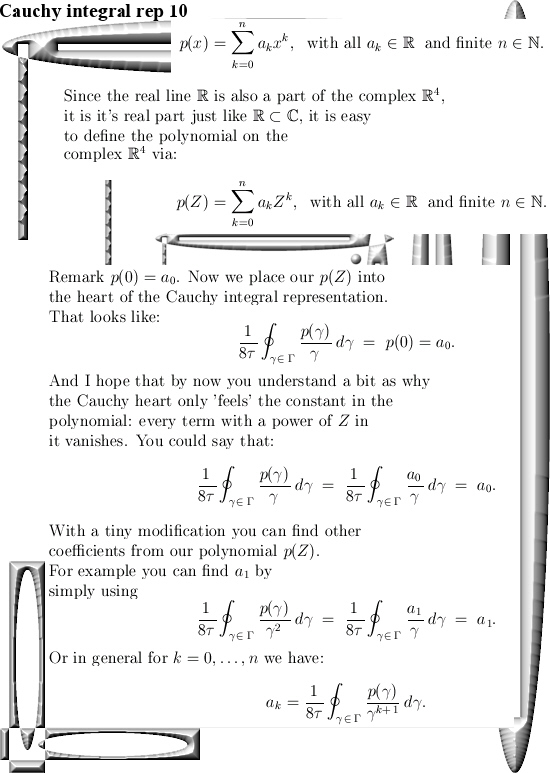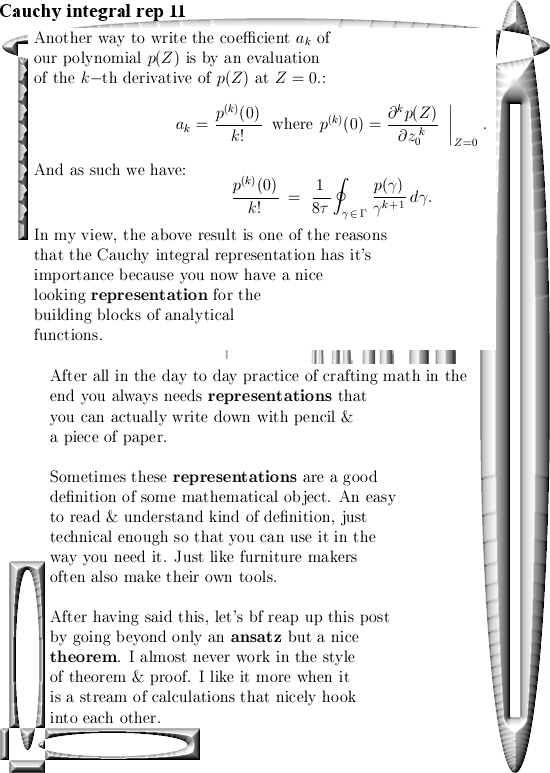I truly hope the math in this update was shallow enough so you can use it in your own path of the math that you like to explore.

End of this post, may be in Part 15 we will finally do a bit more about the diagonalization of 4D complex numbers because that is also a universal way of finding those numbers tau in the different dimensions like the 17D circular numbers & all those other spaces.

Have a nice life or try to get one.

A few numerical results related to the 4D sphere-cone equations using the four coordinate functions of the 4D exponential curve.

This is Part 9 in the basics to the complex 4D numbers. In this post we will check numerically that the 4D exponential curve has it’s values on the 4D unit sphere intersected with a 4D cone that includes all coordinate axes. In 3D space the sphere-cone equations ensure the solution is 1 dimensional like a curve should be. In 4D space the sphere-cone equations are not enough, there is at least one missing equation and those missing equations can be found in the determinant of a matrix representation M(Z) for a 4D complex number Z.

But we haven’t done any determinant stuff yet (because you also need a factorization of the 4D determinant in four variables and that is not a trivial task). So this post does not contain numerical evidence that the determinant is always one on the entire exponential curve.

If you want to compare this post to the same stuff in the complex plane:
In the complex plane the sphere-cone equation is given by x^2 + y^1 = 1 (that is the unit circle) and if you read this you probably know that f(t) = e^{it} = cos t + i sin t.

You can numerically check this by adding the squares of the sine and cosine for all t in one period and that is all we do in this post. Only it is in 4D space and not in the two dimensional complex plane…

This post is seven pictures long (all of the usual size 550 x 775).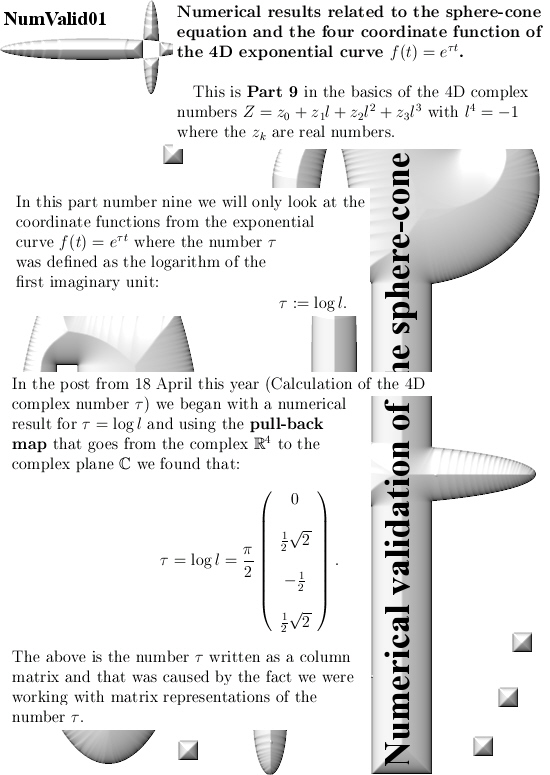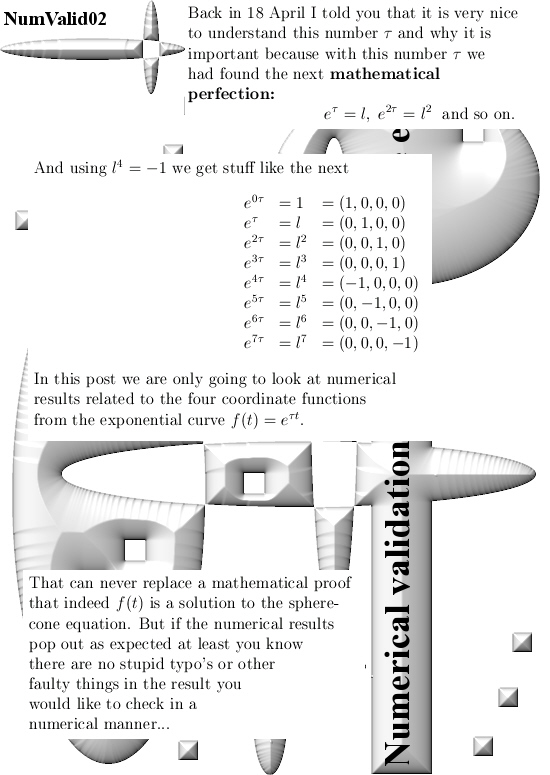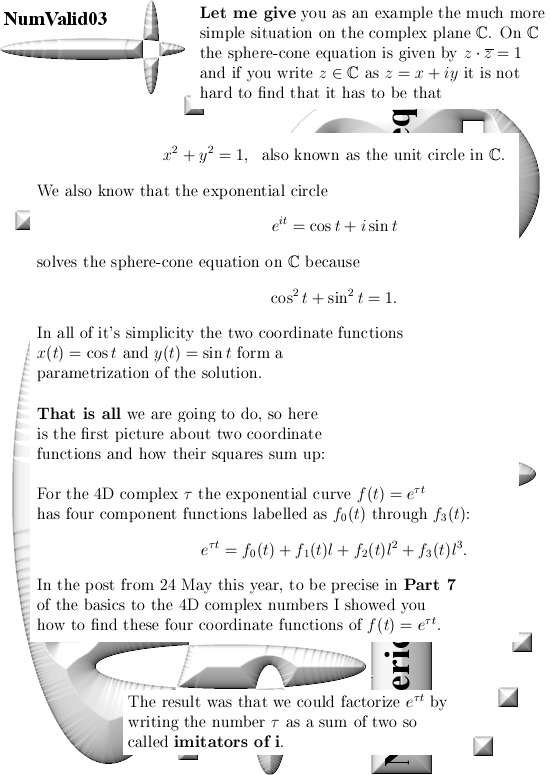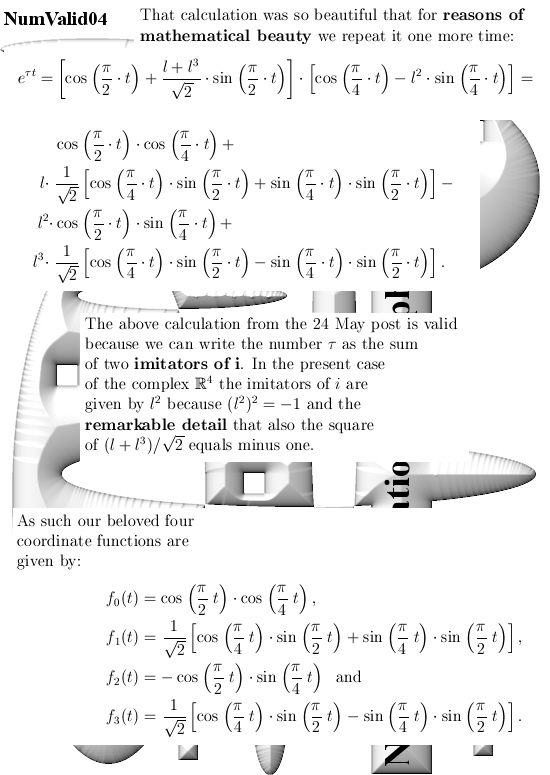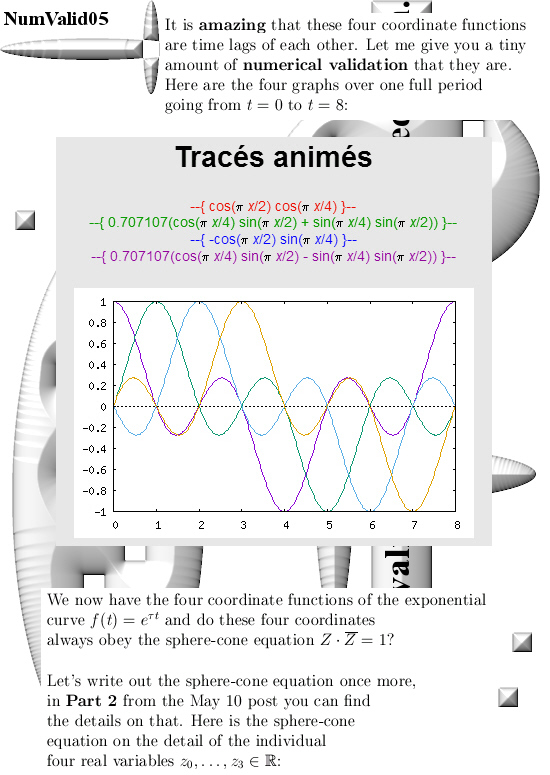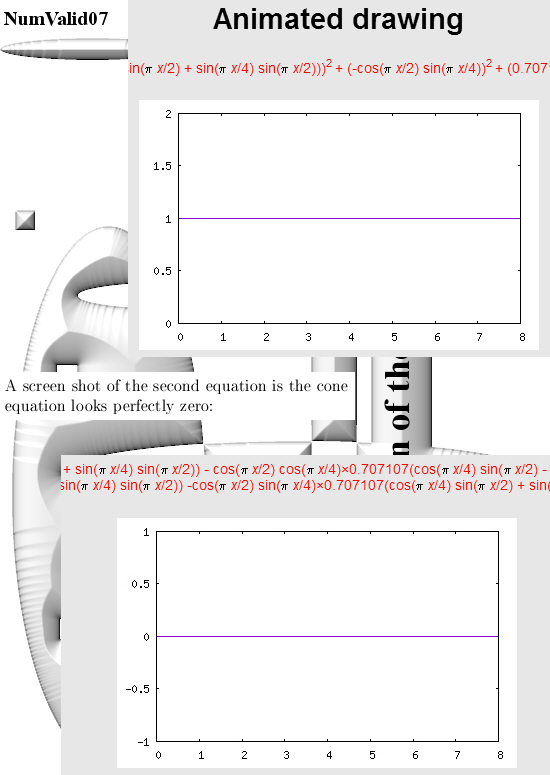All graphs in this post are made with the applets as found on:

For the two graphs from above look for ‘animated drawing’ choose the 2D explicit curves option. There you must use the variable x instead of time t.

Here is the stuff you can place in for the sphere equation:

(cos(pi*x/2)*cos(pi*x/4))^2 +
(0.707107*(cos(pi*x/4)*sin(pi*x/2) + sin(pi*x/4)*sin(pi*x/2)))^2 +
(-cos(pi*x/2)*sin(pi*x/4))^2 +
(0.707107*(cos(pi*x/4)*sin(pi*x/2) – sin(pi*x/4)*sin(pi*x/2)))^2

If you just ‘cut & paste’ it should work fine…
That should save you some typo’s along the way

Ok, that is what I had to say on this numerical detail.

Calculation of the four coordinate functions for the 4D exponential curve (complex version).

Like promised in this post I will show you in the greatest detail possible how to find those rather difficult looking four coordinate functions.

I had thought about crafting these four coordinate functions before but the method I had in mind was rather labor some so I balked a bit at that. Not that I am lazy but I also had to work on the basics for the 4D complex numbers like in the last posts…

So one day I decided to look into the specific details of what I name ‘imitators of the number i’ and I was very surprised by their behavior. As a matter of fact these imitators imitate i soo good that you can make exponential circles of them.
And I wrote down the two exponential circles, I looked at them and realized you can factorize the 4D exponential curve with it and as such you will get the four coordinate functions…

That was all, at some point in time on some day I just decided to look at the imitators of the number i from the complex plane and within 5 at most 10 minutes I found a perfect way of calculating these four coordinate functions.

It always amazes me that often a particular calculation takes a short amount of time, like 10 or 20 minutes, and after that you always need hours and hours until you have a nice set of pictures explaining the calculation…

Anyway, this post is five pictures long and as such it contains also Part 6 and 7 of the Basics to the 4D complex numbers.

I hope that in the long run it will be the result in this post that will make 4D complex numbers acceptable to the main stream mathematical community.
But may be once more I am only fooling myself with that, after all back in the year 1991 I was only thinking stuff like ‘If you show them the 3D Cauchy-Riemann equations, they will jump in the air from joy’.
They (the math professors) never jumped from joy, no significant change in brain activity was ever observed by me. So when I write ‘in the long run’ as above, may be I should more think like a geological timescale…
__________

But let’s not complain because once you understand the factorization, it is so beautiful that it is hard to feel angry or whatever what.
Here are the five pictures: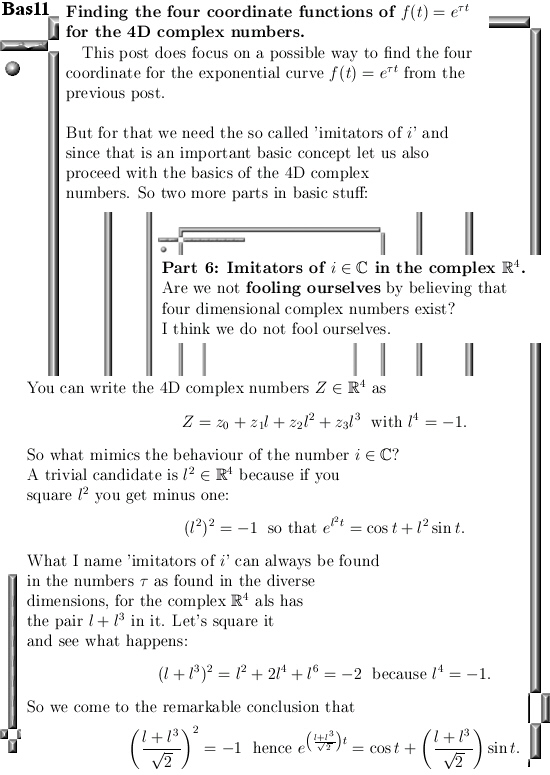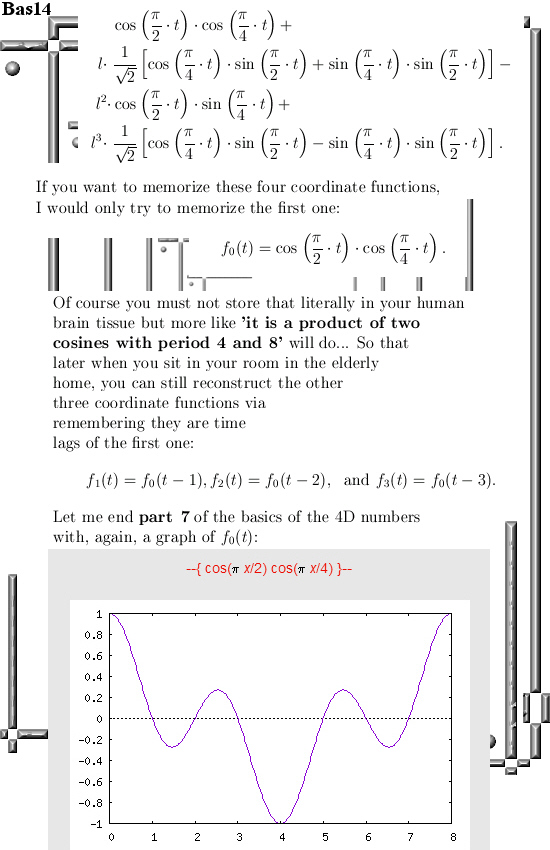Ok, that is how you calculate the four coordinate functions.

The basics of 4D complex numbers.

In the previous post on 4D complex numbers I went a little bit philosophical with asking if these form of crafting a 4D number system is not some advanced way of fooling yourself because your new 4D thing is just a complex plane in disguise…

And I said let’s first craft the Cauchy-Riemann equations for the 4D complex numbers, that might bring a little bit more courage and making us a little bit less hesitant against accepting the 4D complex numbers.

In this post we also do the CR equations and indeed they say that for functions like f(Z) = Z^2 you can find a derivative f'(Z) = 2Z. So from the viewpoint of differentiation and integration we are in a far better spot compared to the four dimensional quaternions from Hamilton. But the fact that the CR equations can be crafted is because the 4D complex numbers commute, that is XY = YX. And on the quaternions you cannot differentiate properly because they do not commute.

So crafting Cauchy-Riemann equations can be done, but it does not solve the problem of may be you are fooling yourself in a complicated manner. Therefore I also included the four coordinate functions of the exponential 4D curve that we looked at in the previous post.

All math loving folks are invited to find the four coordinate functions for themselves, in the next post we will go through all details. And once you understand the details that say the 4D exponential curve is just a product of two exponential circles as found inside our 4D complex numbers, that will convince you much much more about the existence of our freshly unearthed 4D complex numbers.

Of course the mathematical community will do once more in what they are best: ignore all things Reinko Venema related, look the other way, ask for more funding and so on and so on. In my life and life experiences not one university person has ever made a positive difference, all those people are only occupied with how important they are and that’s it. Being mathematical creative is not very high on the list of priorities over there, only conform to a relatively low standard of ‘common talk’ is acceptable behavior…

After having said that, this post is partitioned into five parts and is 10 pictures long. It is relatively basic and in case that for example you have never looked at matrix representations of complex numbers of any dimension, please give it a good thought.

Because in my file I also encountered a few of those professional math professors that were rather surprised by just how a 3 by 3 matrix looks for 3D complex numbers. How can you find that they asked, but it is fucking elementary linear algebra and sometimes I think these people do not understand what is in their own curriculum…

Ok, here are the 10 pictures covering the basic details of 4D complex numbers: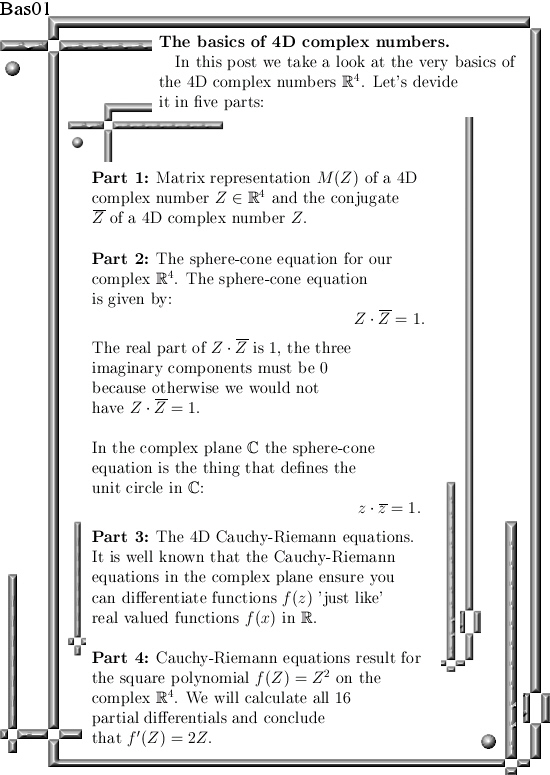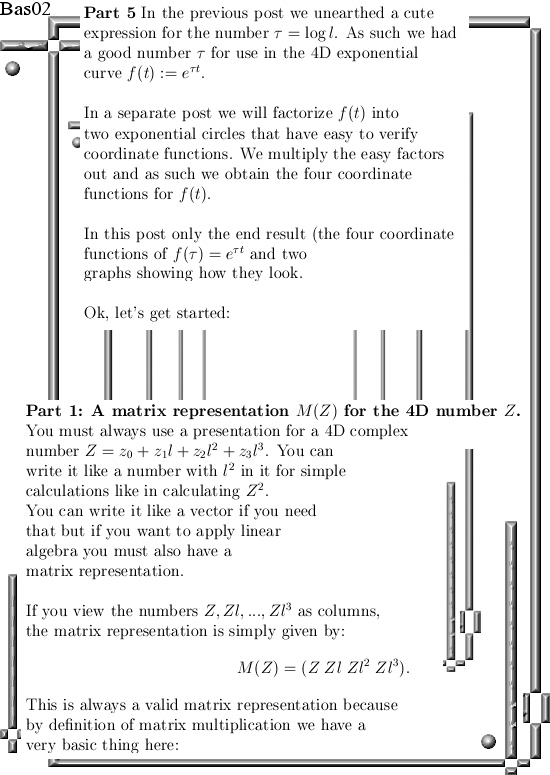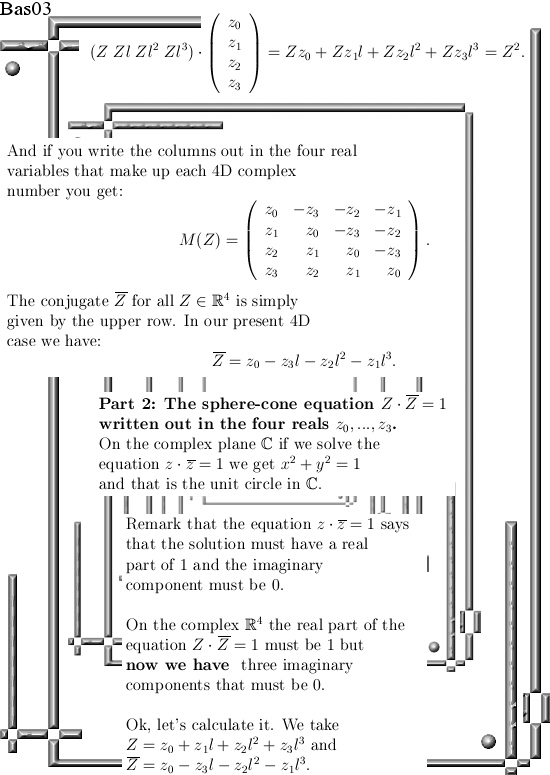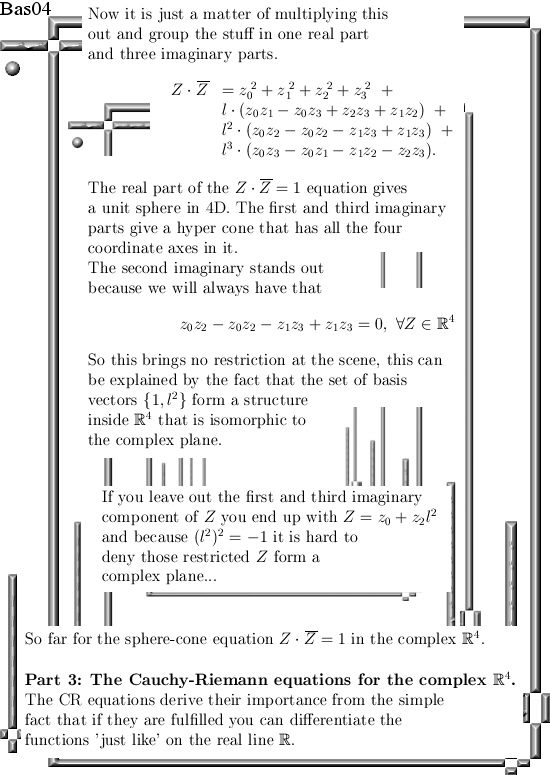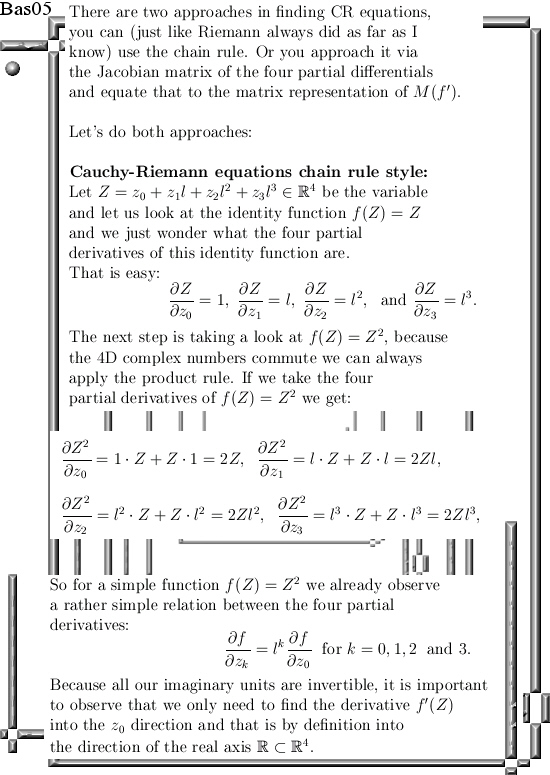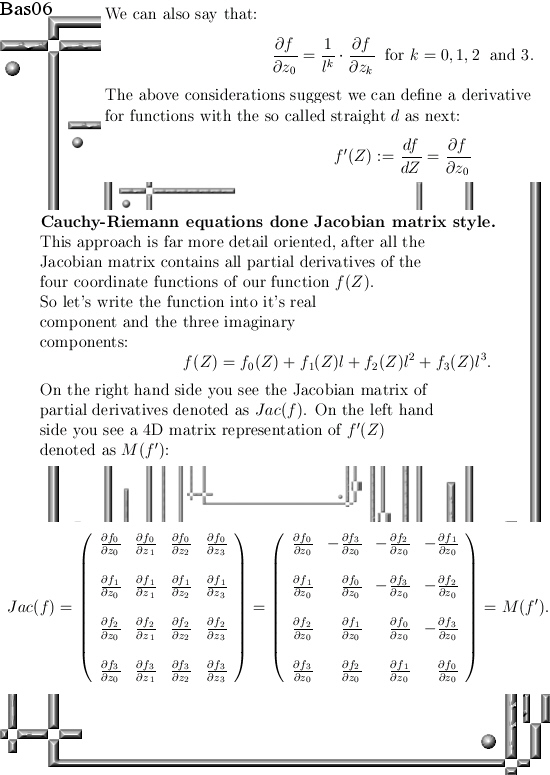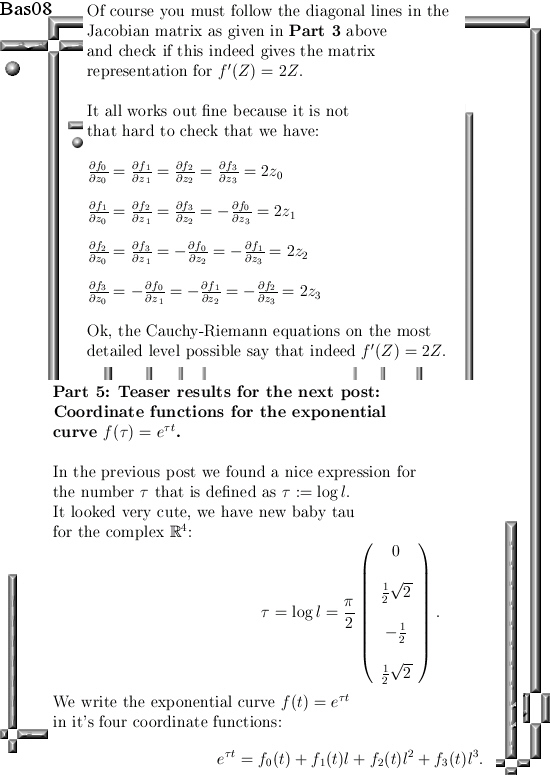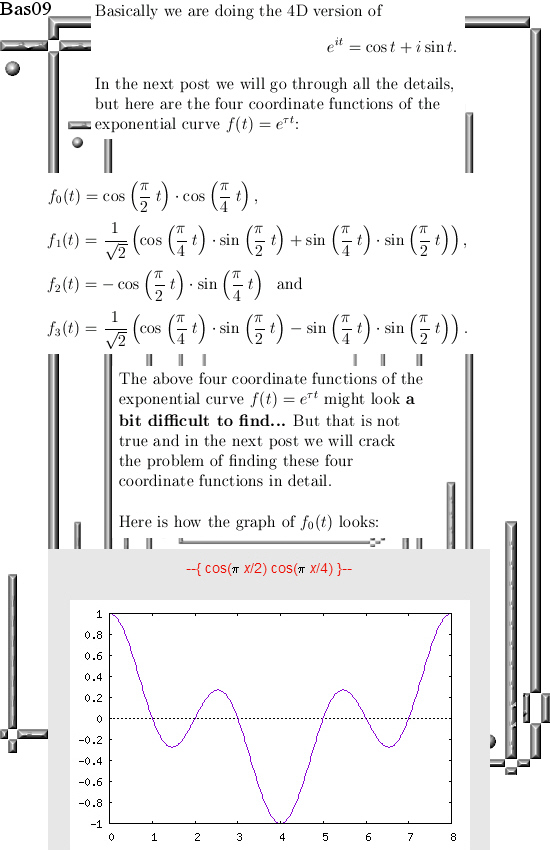Ok, that was the math for this post.

And may be I am coming a bit too hard on the professional math professors. After all they must give lectures, they must attend meetings where all kinds of important stuff has to be discussed until everybody is exhausted, they must be available for students with the questions and problems they have, they must do this and must do that.

At the end of the day, or at the end of the working week, how much hours could they do in free thinking? Not that much I just guess…

Let’s leave it with that, see you in the next post.

A nice teaser picture about 4D coordinate functions of the 4D exponential curve (complex version).

Lately I have been working on the next post about the basics of the 4D complex numbers. You simply need those basics like matrix representations because later on when you throw in some 4D Cauchy-Riemann equations, it is very handy to have a good matrix representation for the stuff involved.

The next post covering the basics had five parts, let’s not dive in all kinds of math details right now but go straight to part five with the four coordinate functions of the 4D exponential curve: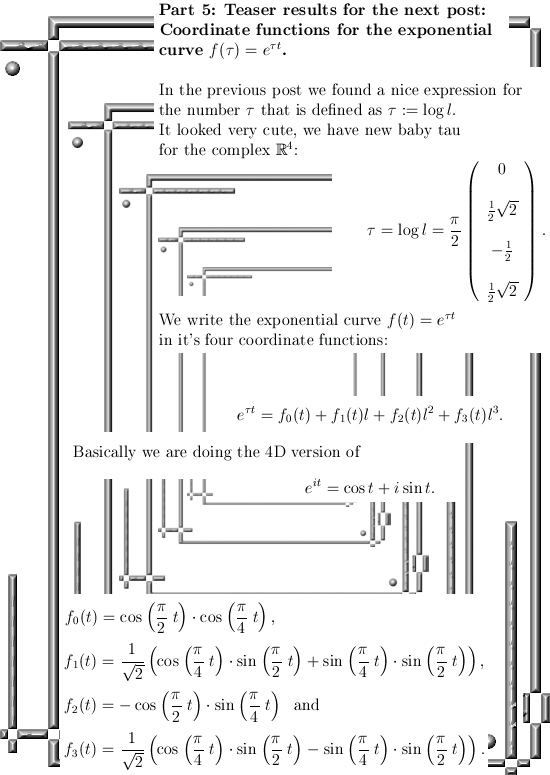These four coordinate functions are also time lags of each other.

This new baby number tau keeps on looking cute…

Let me leave it with that, till updates.

Calculation of the 4D complex number tau.

It is about high time for a new post, now some time ago I proposed looking at those old classical equations like the heat and wave equation and compare that to the Schrödinger equation. But I spilled some food on my notes and threw it away, anyway everybody can look it up for themselves; what often is referred to as the Schrödinger equation looks much more like the heat equation and not like the classical wave equation…

Why this is I don’t know.

This post is a continuation from the 26 Feb post that I wrote after viewing a video from Gerard ‘t Hooft. At the end of the 26 Feb post I showed you the numerical values for the  logarithm of the 4D number tau. This tau in any higher dimensional number system (or a differential algebra in case you precious snowflake can only handle the complex plane and the quaternions) is always important to find.

Informally said, the number tau is the logarithm of the very first imaginary component that has a determinant of 1. For example on the complex plane we have only 1 imaginary component usually denoted as i. Complex numbers can also be written as 2 by 2 matrices and as such the matrix representation of i has a determinant of 1.
And it is a well known result that log i = i pi/2, implicit the physics professors use that every day of every year. Anytime they talk about a phase shift they always use this in the context of multiplication in the complex plane by some number from the unit circle in the complex plane.

In this post, for the very first time after being extremely hesitant in using dimensions that are not a prime number, we go to 4D real space. Remark that 4 is not a prime number because it has a prime factorization of 2 times 2.

Why is that making me hesitant?
That is simple to explain: If you can find the number i from the complex plane into my freshly crafted 4D complex number system, it could very well be this breaks down to only the complex plane. In that case you have made a fake generalization of the 2D complex numbers.

So I have always been very hesitant but I have overcome this hesitation a little bit in the last weeks because it is almost impossible using the complex plane only to calculate the number tau in the four dimensional complex space…

May be in a future post we can look a bit deeper in this danger; if also Cauchy-Riemann equations are satisfied in four real variables, that would bring a bit more courage to further study of the 4D complex number system.

__________

After the introduction blah blah words I can say the 4D tau looks very beautiful. That alone brings some piece of mind. I avoided all mathematical rigor, no ant fucking but just use numerical results and turn them into analytical stuff.

That is justified by the fact that Gerard is a physics professor and as we know from experience math rigor is not very high on the list or priorities over there…

That is forgiven of course because the human brain and putting mathematical rigor on the first place is the perfect way of making no progress at all. In other sciences math should be used as a tool coming from a toolbox of reliable math tools.

__________

This post is seven pictures long, all are 550 by 775 pixels in size except for the last one that I had to make a little bit longer because otherwise you could not see that cute baby tau in the 4D complex space.

Here we go: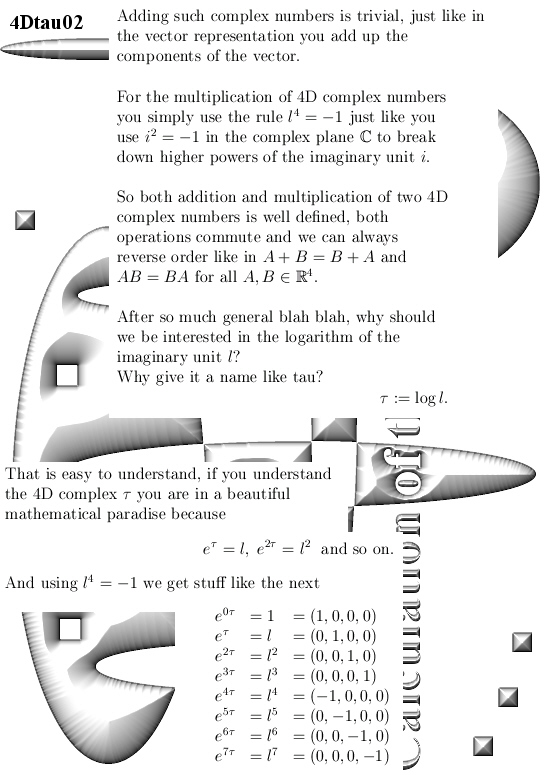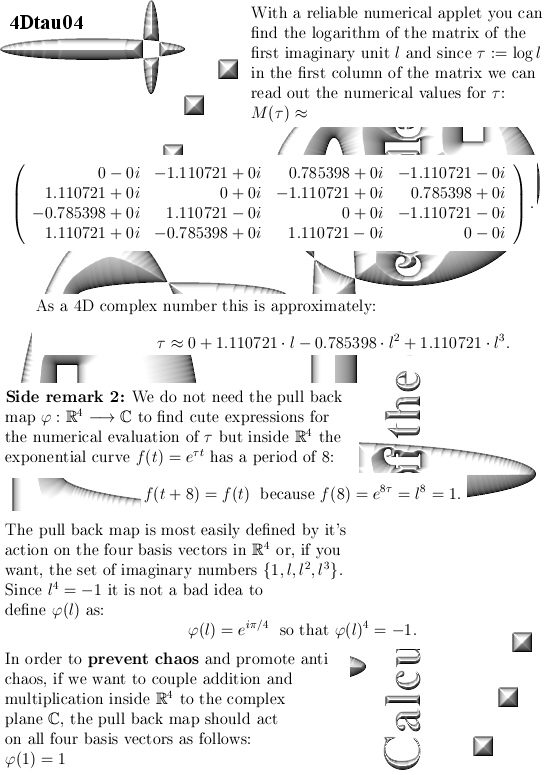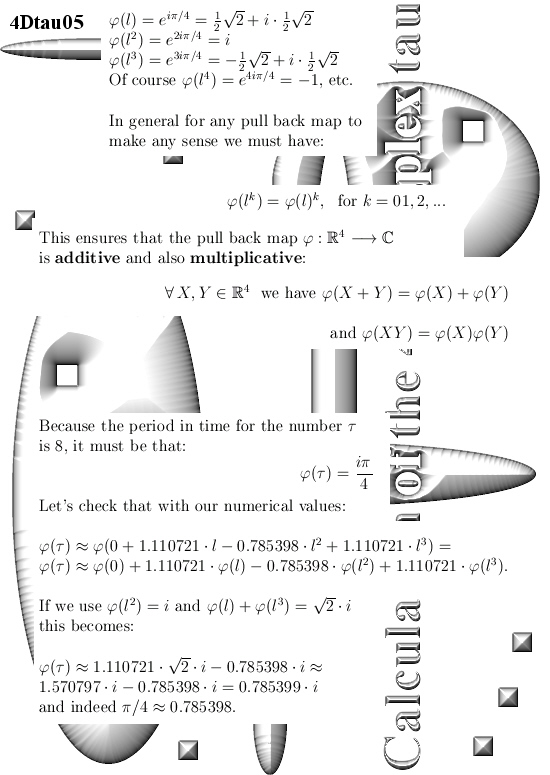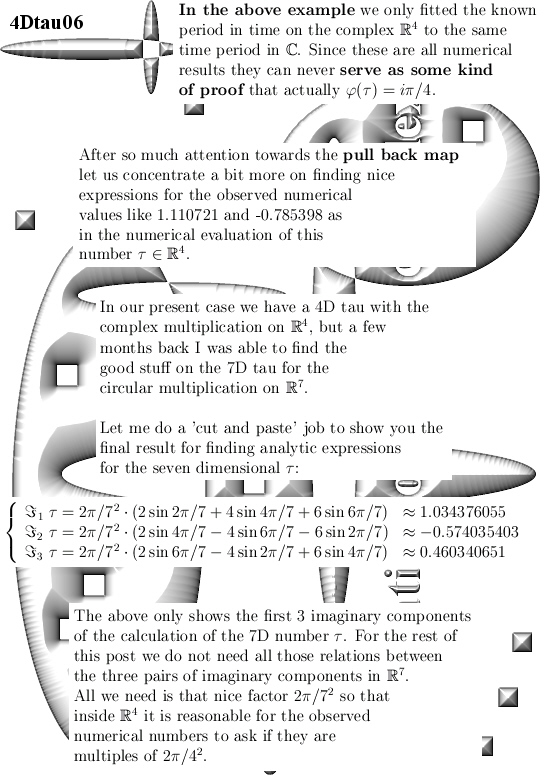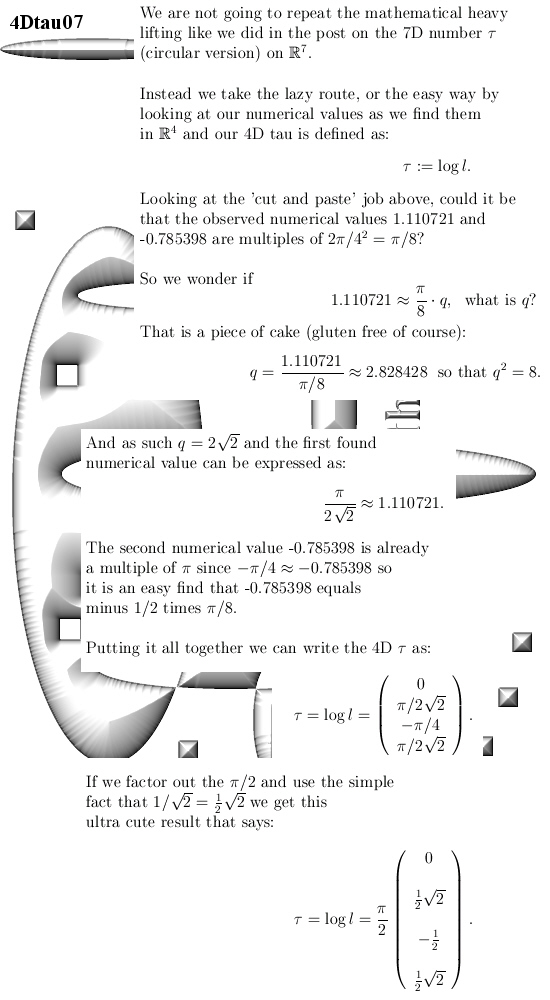Just take your time and look at this ultra cute number tau.

It is very very hard to stay inside the complex plane, of course the use of 4 by 4 matrices is also forbidden, and still find this result…

I am still hesitant about using dimensions that are not prime numbers, but this is a first result that is not bad.

End of this post.

I am innocent, I did not do it. I just found the numbers tau in the Schrödinger equation your honour…

Judge: But you were caught red handed placing the number tau in a Schrödinger equation while you do not qualify for being a member of the most bright and enlightened persons in our society: The PHYSISCS PROFESSORS.

Reinko: But Judge I can explain, it was that evil guy that Gerard ‘t Hooft who did it. I can prove that because it is on video.

Judge: Yes you already told that into the statements you made after arrest by the police. So we took the freedom and ask Mr. Gerard ‘t Hooft himself about the evil you have done with molesting the Schödinger equation. Mr. ‘t Hoofd said it had to be Hermitian and although I do not know what that means he said that by using anti-Hermitian matrices you, Reinko Venema, you are nothing more as some sadistic pedophile piece of shit.

Reinko: But judge, it is not Hermitian, that is only a trick. You see if you multiply it by the number 1 like 1 = – i squared you see it is not Hermitian.

Judge: Do you think we get complex analysis in law school? We don’t, we asked some experts and all agreed that Gerard is right and you are wrong and right now rewarded by your own evil deeds to 75 years in prison in a maximum security facility.

__________

After this somewhat strange introduction I repeat I was innocent. I was just looking at a video of a guy that is just like me old and boring.

And that guy, Gerard ‘t Hooft, was able to give me three nice punches in the face.
That is what this post is about; Three punches in the face as delivered by Gerard.

It is the very first time I observe professional physics professors using the number tau while claiming the stuff has to be Hermitian to make any sense.

I was devastated because in my little world of mathematics it had to be anti Hermitian so at a first glimpse it looks like a simple shootout between Gerard and me: Only one can be right…

Let me first show you the Youtube video where right at the start Gerard succeeds to bring my small sack of human brain tissue into an exited state and after that I am rewarded with finding the number tau into the famous Schrödinger equation.

Let me also temper the enthousiasm a little bit because at present date 26 Feb in the year 2018 I only know of one example where three quantum states are rotated into each other:
That is the transport of the color charges as it is found on the quarks inside the proton and neutron…

Here is the video, after that the nine pictures that make up the mathematical core of this new post:

Gerard ‘t Hooft – How Quantum Mechanics Modifies the Space-Time of a Black Hole (QM90)

Let me spare you a discussion on the entire video but only look at what you can find on the very introduction as shown above because all of the three punches at my face are already found there.

Here are the nine pictures for this new post: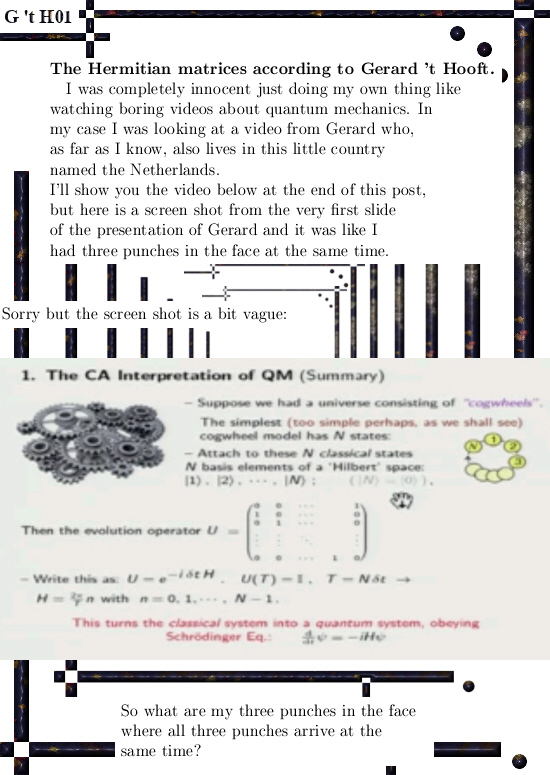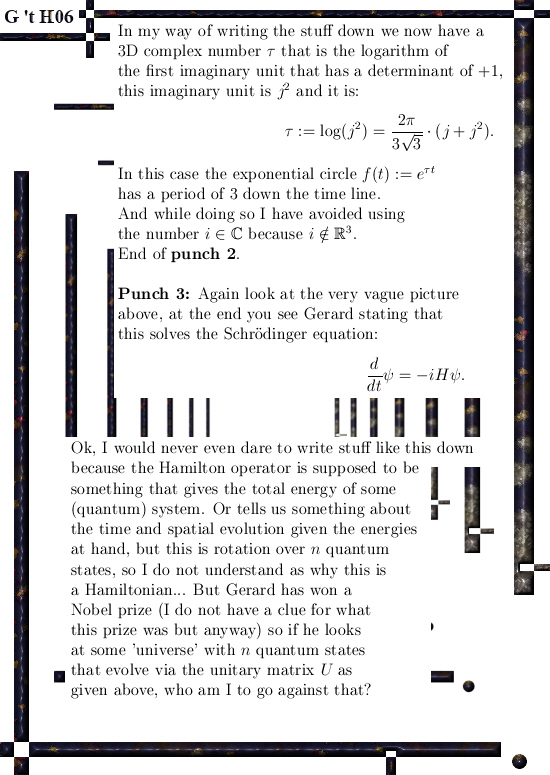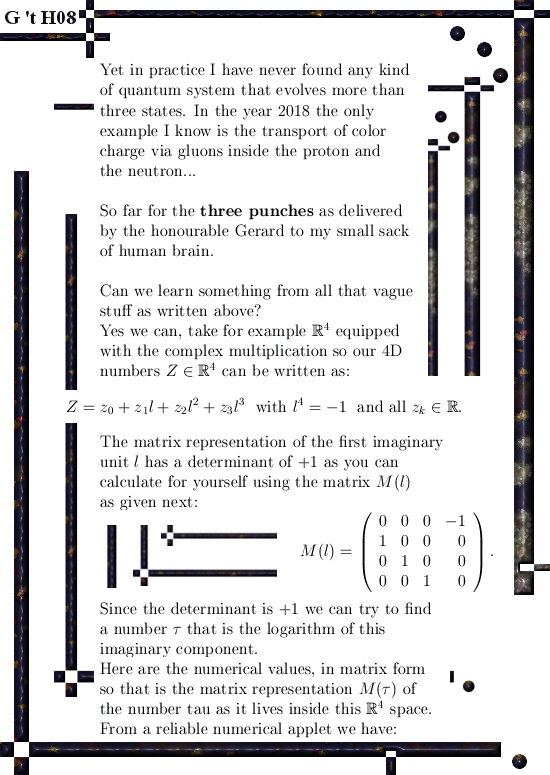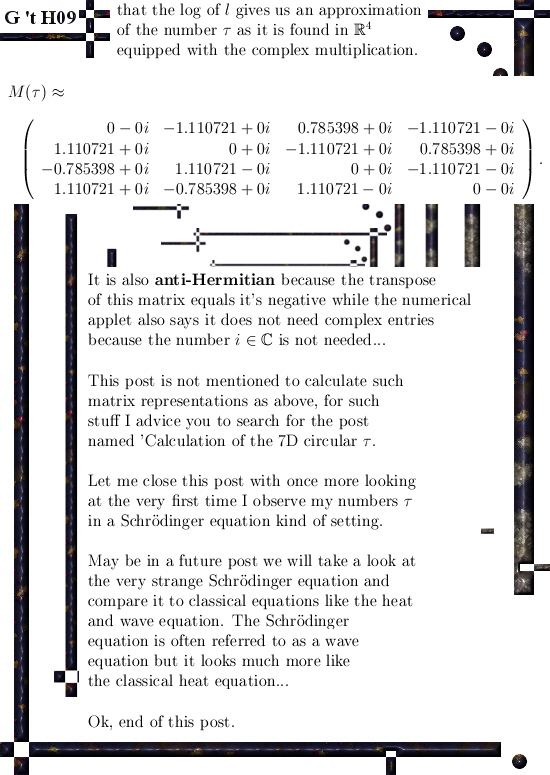For readers who have found themselves lost on what a Hermitian matrix is, here is a wiki:

Hermitian matrix
https://en.wikipedia.org/wiki/Hermitian_matrix

And for readers who have found themselves lost on finding an ‘analytic handle’ about how to calculate matrices like in picture 09, a good starter would be about the calculation of the 7D number tau:

An important calculation of the 7D number tau (circular version).

That’s it, till updates.